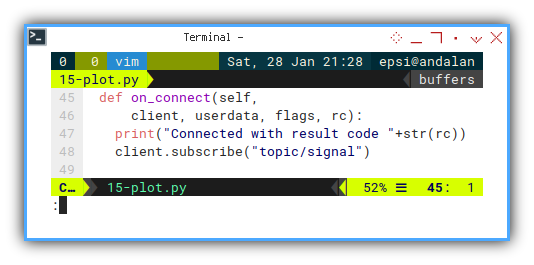# Python - MQTT - Realtime Plot

Python Monitoring Article Series Test

Python - Panda Plot

Python - MQTT Plot

Python - Rich Live

Table of Content
Where to Discuss?

Local Group

### Preface

Goal: Realtime data plot using Pandas.

We plot realtime data for the first time. There is issue, such as focus stealing. We can deal with this with low level matplotlib.

### 7: Realtime Plotting Using Simple Data

Before we go into complex plotting, we have to walk the walk, and plot the plot, run the script.

#### Python Source Code

I recommend you to get an overview with reading the source. So you won’t get lost along the way.

#### Required Package

Interpolate from SciPy

Smoothing our realtime data is easy with SciPy.

``````import paho.mqtt.client as mqtt
import matplotlib
import matplotlib.pyplot as plt
import numpy as np
import time

from scipy import interpolate``````#### Skeleton

Looks like there’s no complex stuff. But in details, some method are longer than usual.

``````class mqSubscriber:
def __init__(self):
def chart_setup(self):
def update_line(self, new_num):
def on_connect(self, client, userdata, flags, rc):
def on_message(self, client, userdata, msg):
def main(self):``````#### Constructor

We need to separate chart setup and initial data.

``````class mqSubscriber:
def __init__(self):
# save initial parameter
self.index = 0
self.chart_setup()``````#### Setting Up Chart

Do not forget to setup the chart.

``````  def chart_setup(self):
self.xdata = []
self.ydata = []

self.fig, self.axes = plt.subplots()
self.line, = self.axes.plot(
self.xdata, self.ydata, 'r-')

self.fig.canvas.mpl_connect(
'close_event', exit)``````We will use `self.axes` and `self.lines` in our class method.

#### Main Method

Just very ordinary `MQTT`.

``````  def main(self):
plt.ion()
plt.show()

client = mqtt.Client()
client.connect("localhost",1883,60)

client.on_connect = self.on_connect
client.on_message = self.on_message

client.loop_forever()``````Except that we have this two lines:

``````    plt.ion()
plt.show()``````

We should set the interactive mode on in realtime plot, by calling `plt.ion()`.

#### Event Handler: On Connect

Nothing special. The same as official documentation.

``````  def on_connect(self,
client, userdata, flags, rc):
print("Connected with result code "+str(rc))
client.subscribe("topic/signal")``````#### Event Handler: On Message

We need to update the line. I put the detail on its own method.

``````  def on_message(self, client, userdata, msg):

self.update_line(float_num)``````

Since the data change over time passes, we need to update the limit

``````    # recompute the ax.dataLim
# update ax.viewLim using the new dataLim
self.axes.relim()
self.axes.autoscale_view()``````This is why I set `self.axes` on the chart setup.

#### Displaying Chart

Plotting Issue

All good, the data displayed correctly. Except that when I call `plt.show()`. The window steal focus. I can’t evenm type on my terminal, because the focus always go back to matplotlib chart.

``````  def on_message(self, client, userdata, msg):
...

# issue: steal focus
plt.draw()

self.fig.canvas.draw_idle()
self.fig.canvas.start_event_loop(0.001)

time.sleep(0.1)``````This is why I set `self.fig` on the first place.

I must admit, that I do no really understand about, what’s really going on here. But this works for me. Maybe I will go deep into low level matplotlib some other time.

#### Interpolation

Interpolation can be done this way:

``````      n = len(self.ydata)
tck = interpolate.splrep(
self.xdata, self.ydata, s=0)
xfit = np.arange(0, n-1, np.pi/50)
yfit = interpolate.splev(xfit, tck, der=0)``````

But we should aware that we can’t interpolate data, if the number of data is insufficient.

The result of interpolation can be displayed in below figure.#### Updating Line

MQTT Message Handler

The complete method is here below. Afterr get the right `xfit` and `yfit` series, we can update the data series into matplotlib axes.

``````  def update_line(self, new_num):
self.index += 1
self.xdata.append(self.index)
self.ydata.append(new_num)

if self.index <= 3:
self.line.set_xdata(self.xdata)
self.line.set_ydata(self.ydata)
else:
n = len(self.ydata)
tck = interpolate.splrep(
self.xdata, self.ydata, s=0)
xfit = np.arange(0, n-1, np.pi/50)
yfit = interpolate.splev(xfit, tck, der=0)

self.line.set_xdata(xfit)
self.line.set_ydata(yfit)``````This is why I set `self.line` from the very beginning.

#### Execute: Run

We can humbly run the class in peace.

``````mqSub = mqSubscriber()
mqSub.main()``````#### Chart Preview

It is a good time to run the code above.

This chart video below, should help you figure out, the result of code above.

As you can see the start point is stuck there, we will need to enhance the plot, so it looks like moving chart.

### What is Next 🤔?

Simple realtime plotting achieved,but the real challenge is smoothing time series using dataframe. We have already solve smoothing time series in previous article, we still need to combine the trick with above plot, and also we need to make pretty chart.

This is the minimum viable prototype that we want to achieve.

Consider continue reading [ Python - MQTT - Smooth Timeseries Plot ].